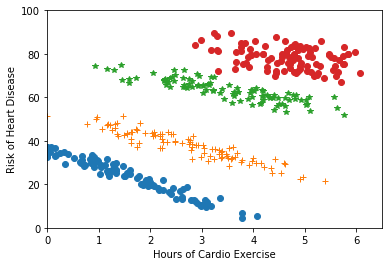## Bands of Bands

Regré Shun runs a gym and collected data about members of an exercise program. They’ve heard that the number of hours you exercise is linearly related to the risk of heart disease in the future, so they decide to do a quick linear regression problem (i.e., order = 1). Their output $\theta$ and $\theta_0$, however, seem to imply that the more hours one exercises, the higher the risk of heart disease. This is confusing since Regré always thought exercise was good for you! So, they call you up for help and you ask to look at the data. Here’s what you see:4A) What do you observe about this data? Did Regré calculate their model correctly? Does exercise increase your risk of disease? 4B) When you look carefully, you can see that there appear to be “bands” in the data (see figure below). It turns out these bands correspond to age groups. How might that affect heart disease risk?4C) Knowing this, how could you change your model? Would using multiple models help? If you wanted to use a single model, what could you do? (It's okay if you don't have perfect answers to these questions! The checkoff is meant as an avenue to discuss!) 4D) In this case, Regré Shun had the idea to look more carefully at the data since it didn't agree with their intuition. What might have happened if they had run a regression analysis on some data, and the results did agree with their intuition, but their intuition was wrong? What if they had no intuition about their data or a way to visualize it? Does your answer have any implications for using machine learning tools in practice? Are there situations where we care more about these questions than in others? Food for Thought
The phenomenon illustrated above is called “Simpson’s Paradox”. It is a statistical phenomenon where a trend appears in several different subgroups in data, but disappears -- and sometimes reverses -- when the subgroups are combined. Here's what the data looks like when viewed in three-dimensions -- note we can still fit a linear model to this (a plane)!